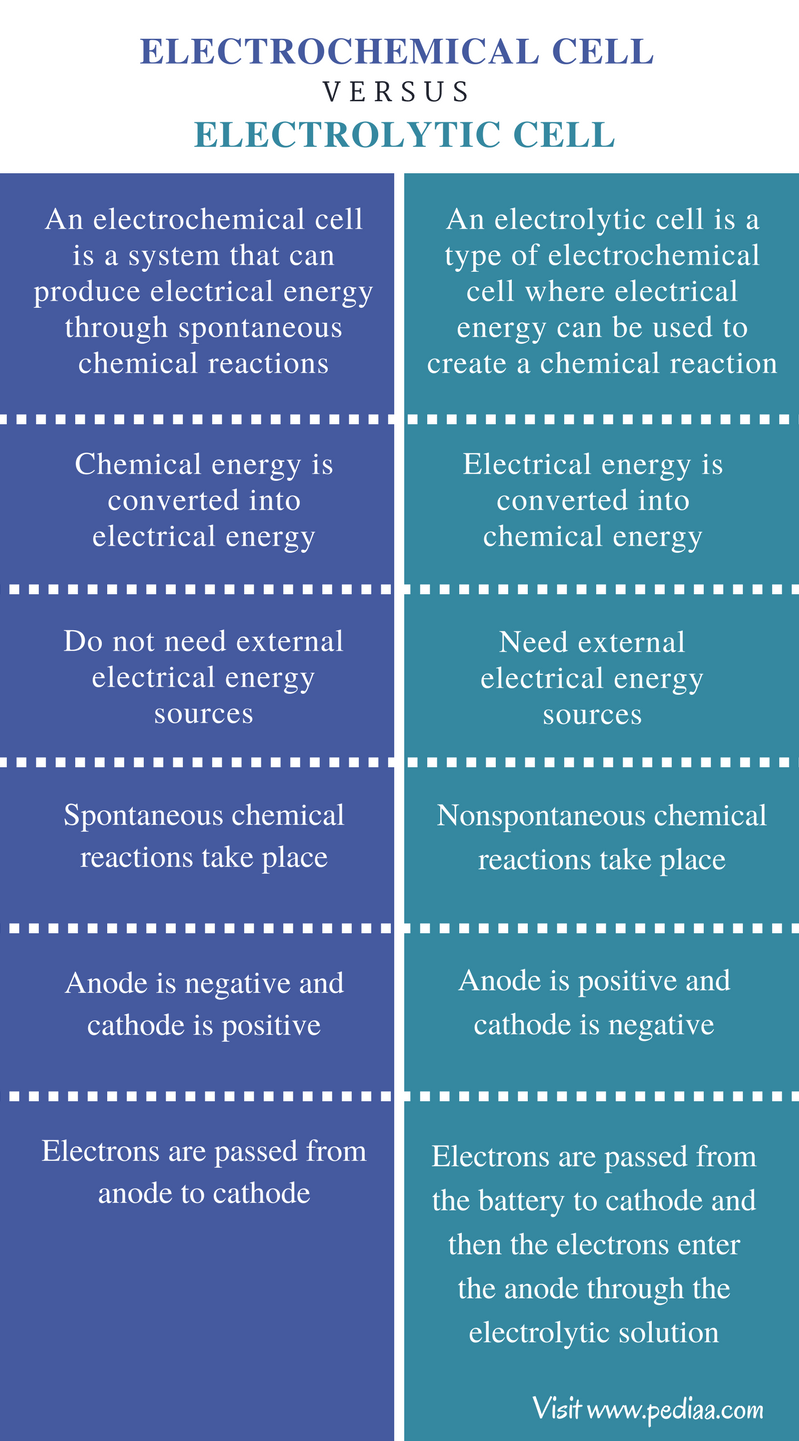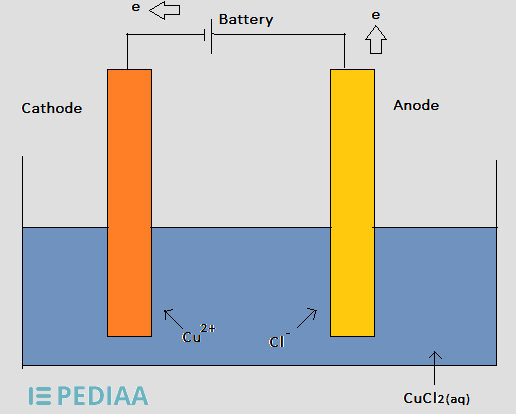# Difference Between Electrochemical Cell and Electrolytic Cell

## Main Difference – Electrochemical Cell vs Electrolytic Cell

Electrochemistry includes the study of the movement of electrons in systems where chemical processes take place. Here, chemical reactions can be used to generate an electrical current or an electrical current can be used to facilitate a nonspontaneous chemical reaction to occur. In both ways, the conversion of electrical energy into chemical energy or the opposite of that will occur. The systems where these conversions take place are known as cells or, more precisely, electrochemical cells. There are two types of electrochemical cells known as voltaic cells and electrolytic cells. The main difference between electrochemical cell and electrolytic cell is that electrochemical cell does not need any external current for operation whereas electrolytic cells need external current to operate.

### Key Areas Covered

1. What is Electrochemical Cell
– Definition, Properties, How it Works
2. What is Electrolytic Cell
– Definition, Properties, How it Works
3. What is the Difference Between Electrochemical Cell and Electrolytic Cell
– Comparison of Key Differences

Key Terms: Anode, Cathode, Electrochemical Cell, Electrolysis, Electrolytic Cell, Galvanic Cell, Oxidation, Reduction, Voltaic Cell## What is an Electrochemical Cell

An electrochemical cell is a system that can produce electrical energy through spontaneous chemical reactions. The chemical reactions that are involved in this process are called redox reactions. Redox reactions occur through transferring of electrons between chemical species. A redox reaction includes two half-reactions: oxidation reaction and reduction reaction. The oxidation reaction always releases electrons to the system whereas the reduction reaction takes electrons from the system. Therefore, the two half-reactions occur simultaneously.

Electrochemical cells are found in two types, as voltaic (galvanic) cells and electrolytic cells. An electrochemical cell is composed of two half cells. The half-reactions occur in two half cells. The chemical reactions that take place in that cell cause the build up of a potential difference between two half cells.

A half-cell should be composed of an electrode and an electrolyte. Therefore, a complete electrochemical cell is composed of two electrodes and two electrolytes; sometimes, the two half cells may use the same electrolyte. If there are two different electrolytes used, then a salt bridge should be used in order to keep the contact between the electrolytes. It is done by making a passage to transfer ions through the salt bridge. The electrons flow from one-half cell to the other through an external circuit. The two electrodes are called anode and cathode.

The oxidation and reduction reactions occur in two electrodes separately. The oxidation reaction occurs in the anode whereas the reduction reaction occurs in the cathode. Therefore, electrons are produced in the anode and they move from anode to cathode through the external circuit. The salt bridge helps to maintain the system neutral (electrically) by transferring ions through it in order to balance the electrical charges.

Let’s consider the following electrochemical cell.Figure 1: Electrochemical Cell

Here, the anode is the Zn (Zinc) electrode and the cathode is the Cu (Copper) electrode. The oxidation reaction occurs in the Zn electrode. There, the metallic Zn is oxidized into Zn+2 ions. The released electrons are passed through the external wire. Produced Zn+2 ions are released into the solution. Therefore, Zn electrode will be dissolved with the time. The reduction reaction occurs near the cathode. The cathode is a Cu electrode. There, the electrons coming from the external circuit are taken by the Cu2+ ions in the solution and are reduced into Cu metal. Therefore, the mass of the Cu electrode will be increased with time. The electron flow through the external wire can be measured as the electrical current produced from the redox reaction. This is the typical structure of an electrochemical cell.

### Reactions

• Reaction in the Anode (oxidation)

Zn(s)         →           Zn+2(aq)     +      2e

• Reaction in the Cathode (reduction)

Cu+2(aq)      +     2e              →           Cu(s)

## What is an Electrolytic Cell

An electrolytic cell is a type of electrochemical cell in which electrical energy can be used to cause a chemical reaction to occur. In other words, the electrical energy should be supplied from an outside source. Then a nonspontaneous reaction can be initiated. Electrolytic cells are most commonly used for electrolysis of compounds.

An electrolytic cell is also composed of solid metals as electrodes. There are two electrodes connected to an external circuit. One electrode acts as the anode whereas other one acts as the cathode. The oxidation reaction will occur in the anode and the reduction reaction will occur in the cathode.

The external electrical energy supply (from the battery connected to the two electrodes) provide an electron flow through the cathode. These electrons then enter the electrolytic solution. Then, the cations in the solution gather around the cathode and obtain electrons that are coming through the cathode. Therefore, these cations are reduced at the cathode. The electrons in the cathode repel anions in the solution. These anions migrate towards the anode. There, these anions release electrons and get oxidized. Therefore, the anode has a positive charge and cathode has a negative charge.

Let us consider the following example.Figure 2: The Electrolysis of Copper Chloride Solution

In the above electrolytic cell, the battery provides electrons to the cathode and Cu+2 ions gather around the cathode to take the electrons from the cathode. Then the Cu+2 ions are reduced to Cu metal and are deposited on the cathode. Then Cl ions migrate towards the anode and release the excess electron they have. There, the oxidation of Cl occurs forming Cl2(g).

### Reactions

• Reaction in the Anode (oxidation)

2Cl(aq)         →           Cl2(g)     +      2e

• Reaction in the Cathode (reduction)

Cu+2(aq)      +     2e              →           Cu(s)

## Difference Between Electrochemical Cell and Electrolytic Cell

### Definition

Electrochemical Cell: An electrochemical cell is a system that can produce electrical energy through spontaneous chemical reactions.

Electrolytic Cell: An electrolytic cell is a type of electrochemical cell where electrical energy can be used to create a chemical reaction.

### Energy Conversion

Electrochemical Cell: In electrochemical cell, chemical energy is converted into electrical energy.

Electrolytic Cell: In electrolytic cell, electrical energy is converted into chemical energy.

### External Current

Electrochemical Cell: Electrochemical cells do not need external electrical energy sources.

Electrolytic Cell: Electrolytic cells need external electrical energy sources.

### Chemical Reactions

Electrochemical Cell: In electrochemical cells, spontaneous chemical reactions take place.

Electrolytic Cell: In electrolytic cells, nonspontaneous chemical reactions take place.

### Electrodes

Electrochemical Cell: In an electrochemical cell, the anode is negative and the cathode is positive.

Electrolytic Cell: In an electrolytic cell, the anode is positive and the cathode is negative.

### Electron Movement

Electrochemical Cell: Electrons are passed from anode to cathode in electrochemical cells.

Electrolytic Cell: Electrons are passed from the battery to cathode and then the electrons enter the anode through the electrolytic solution in electrolytic cells.

### Conclusion

An electrolytic cell is a type of electrochemical cell. Therefore, the electrolytic cell is composed of all the components that a typical electrochemical cell has. Both electrochemical cells and electrolytic cells involve the circulation of electrons through the system. However, in electrochemical cells, spontaneous chemical reactions take place whereas, nonspontaneous chemical reactions take place in electrolytic cells. This is the difference between electrochemical cell and electrolytic cell.

##### References:

1.” Electrochemical cell.” Wikipedia. Wikimedia Foundation, 24 July 2017. Web. Available here. 26 July 2017.
2.”Electrolytic Cells.” Chemistry LibreTexts. Libretexts, 21 July 2016. Web. Available here. 26 July 2017.

##### Image Courtesy:

1. “Electrochemical cell” by Siyavula Education (CC BY 2.0) via Flickr